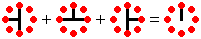# Gerdig Ufo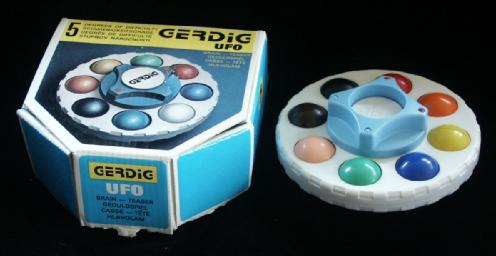There are several puzzles called Ufo. Like most of these, this one also consists of a disc which is split into two layers which can rotate about the central axis. Spaced evenly around this disk are 8 spheres of different colours. These are split into an upper and a lower half, so that when the layers of the disc are turned, one half of each sphere travels with each layer. In the middle of the puzzle is a button that can be pushed up or down. In the simplest difficulty setting, moving the button will turn over one sphere. By turning the central hub before pressing its button, you can choose which sphere is turned over.

Inside the centre hub there is a clever rack and pinion mechanism that turns over a sphere when the button is moved. You can rearrange the pieces of this mechanism so that up to four spheres are turned over simultaneously by the button. This gives five difficulty settings. The spheres that turn over in each setting are:
 1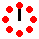One sphere. 2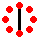Two opposite spheres. 3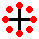Four spheres. 4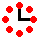Two spheres at 90 degrees to each other. 5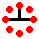Three spheres.

After setting the level, you can set little markers on the hub to show which sides are active.

The various difficulty settings will be treated separately below. The order listed here is that given in the leaflet of the puzzle. In my opinion this is not in increasing order of difficulty.

The Ufo was invented by Gerhard Huncaga, and has patent US 5,370,394 granted on 6 December 1994 and a German patent DE 4106896 granted on 5 March 1991.

If your browser supports JavaScript, then you can play Gerdig Ufo by clicking the link below:

## Level 1.## The number of positions of level 1:

There are 16 pieces, 2 of each colour. They can therefore be arranged in at most 16!/28=81,729,648,000 positions. All these positions are indeed attainable. The orientation of the puzzle is immaterial, so there are actually only about 16!/(28·16) = 5,108,103,000 distinct positions. Some positions are symmetrical under certain rotations of the whole puzzle, so this number is a slight underestimate. The exact number can be calculated using Burnside's Lemma, and this gives 5,108,125,680 distinct positions. The colours in the solution are equivalent however, so there are 8!/16 = 2,520 solutions.
If positions that differ only by recolouring are considered the same, then there is only one solution, but the total number of positions is a bit more than 16!/(28·16·8!), again because some positions exhibit symmetry. Applying Burnside's Lemma in this case is quite tricky, and if I have calculated this correctly, there are actually 130,040 possible essentially distinct positions.

## Solution to level 1:

Phase 1: Solve one sphere.

1. If possible, turn one disk to match up as many pieces as you can.
2. If no pieces can be matched up, push the button and try again.

Phase 2: Solve the rest of the spheres.

1. Choose a colour to solve, and find the two pieces of that colour.
2. If the two pieces are in different disks, then turn over one of them.
3. Turn the hub so that the marker lies at one of the pieces.
4. Turn the disk containing the two pieces until the second piece lies at the marker.
5. Move the button.
6. Turn the disks to bring the two pieces together. Any previously solved spheres will still be intact.
7. Repeat steps a-f until the puzzle is solved.

## Level 2.## The number of positions of level 2:

Pieces on opposite sides of the puzzle always remain so. Therefore such a pair can be considered as if it were a single puzzle piece with two orientations. There are then 8 pieces each with 2 orientations occurring in 4 identical pairs. They can therefore be arranged in at most 8!·28/24 = 645,120 positions. All these positions are indeed attainable. The orientation of the puzzle is immaterial, so there are actually only about 8!·28/(16·24) = 40,320 distinct positions. Some positions are symmetrical under certain rotations of the whole puzzle, so this number is a slight underestimate. The exact number can be calculated using Burnside's Lemma, and this gives 40,332 distinct positions. The colours in the solution are equivalent however, so there are 4!·24/16 = 24 solutions.
If positions that differ only by recolouring are considered the same then, if I have applied Burnside's Lemma correctly, there are actually 262 essentially distinct positions.

## Solution to level 2:

1. Use same method as Level 1 to solve three pairs of opposite spheres.
2. The final pair of opposite spheres might already be solved, but if not then do these steps:
1. Turn the hub so that the markers are at the unsolved spheres.
2. Turn the top layer 1 step clockwise and then press the button.
3. Repeat step 2 three more times.
4. Give the top layer a half turn.

## Level 3.## The number of positions of level 3:

The 16 pieces actually split into 4 sets which each move as a unit. Such a set of 4 pieces can be considered as if they were a single puzzle piece with four orientations. There are then 4 pieces each with 4 orientations occurring in 2 identical pairs. They can therefore be arranged in at most 4!·44/2!2 = 1,536 positions. All these positions are indeed attainable. The orientation of the puzzle is immaterial, so there are actually only 4!·44/(8·2!2) = 192 distinct positions. This number is exact because none of the positions are symmetrical under rotations of the whole puzzle. The order of the colours in the solution does not matter, so there are 4 solutions.

If positions that differ only by recolouring are considered the same then, if counted them correctly, there are actually 26 essentially distinct positions.

## Solution to level 3:

1. If each side has only 4 colours, then press the button.
2. Turn one disk until you either solve the puzzle, or else until the spheres come in identical pairs with the same colours (possibly with some solved spheres).
3. If four spheres are solved and four unsolved then turn one disk two steps.
4. Press the button.
5. If each side has only four colours, then turn one disk one step, and press the button again.
6. Turn one disk until it is solved.

## Level 4.## The number of positions of level 4:

This has the same number of positions as level 1, i.e. there are 5,108,125,680 distinct positions, or 130,040 if positions that differ only by recolouring are considered the same.

## Solution to level 4:

Phase 1: Separate the colours.
This phase puts four pairs of pieces in each disk, so that there are only four colours in each layer.

1. Find a piece in the bottom layer that belongs in the top layer.
2. Find a piece in the top layer that belongs in the bottom layer.
3. Turn the disk to bring these two pieces to the same sphere.
4. Turn the hub so that this sphere is at a marker.
5. Give the top layer a half turn (i.e. four steps).
6. Push the button. This brings the piece from the bottom layer to the top, but does disturb some other pieces from the top.
7. Give the top layer a quarter turn (two steps) so that the aforementioned piece is not at a marker.
8. Push the button again, restoring any previously disturbed pieces.
9. Repeat a-h until each layer has only four colours.

Phase 2: Rearrangement.
This phase arranges the pieces from the top layer into one set of 4 spheres, and those of the bottom layer in the other set.

1. Push the button.
2. Give the hub a half turn (i.e. 4 steps).
3. Push the button again.
4. Turn one disk so that as many spheres as possible are solved.

Phase 3: Solve the spheres.
Remember that the spheres fall into two sets of four, and you can solve these independently from each other as the markers will always be on spheres from the same set.

1. Solve two adjacent spheres from the same set (i.e. two spheres that are 90 degrees apart). This is quite straightforward, and very similar to solving level 1. The main difference is that while you solve a sphere using one of the markers, other pieces will become mixed at the other marker in the same set. Suppose that you hold the hub with one marker at the front and the other at the left, and the pieces in the top layer at the markers have the same colour. The following moves will match them together, without disturbing the sphere at the right:
1. Turn the top layer anti-clockwise a quarter turn (two steps).
2. Press the button.
3. Turn the top layer clockwise a quarter turn.
2. The two remaining spheres will either be already solved (so skip to step d), or have the same two colours. If they have the same colour on top, then turn over one of them (put one marker at one of the spheres, the other marker at a solved sphere, and press the button).
3. Put the markers at the two unsolved spheres, and do these moves:
1. Turn the bottom layer two steps to the right (clockwise when viewed from below).
2. Press the button.
3. Turn the bottom layer back (two steps to the left).
4. Press the button.
5. Turn the top layer two steps to the right (anti-clockwise when viewed from above).
6. Press the button.
7. Turn the top layer back (two steps to the left).
4. Solve the other set of spheres in the same way, using steps a-c.

## Level 5.## The number of positions of level 5:

This has the same number of positions as level 1, i.e. there are 5,108,125,680 distinct positions, or 130,040 if positions that differ only by recolouring are considered the same.

## Solution to level 5:

It is possible to turn a single sphere by turning the hub to bring each of the markers at the sphere in turn, and pressing the button each time. This means that the solution is essentially the same as that of level 1.